# Surface integral

(diff) ← Older revision | Latest revision (diff) | Newer revision → (diff)

An integral over a surface. Let a surfacein the three-dimensional Euclidean space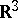, possibly with self-intersections, be specified by the vector representation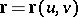(1)

in Cartesian coordinates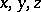, where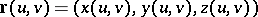is a continuously-differentiable vector function defined on the closureof a two-dimensional Jordan-measurable domainlying in the plane with Cartesian coordinatesand. Letbe the coefficients of the first fundamental form of. If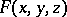is a function defined on, i.e. a function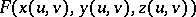, then the following defines the surface integral of the first kind (or the integral over the surface area):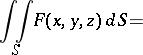(2)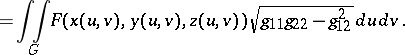This definition is independent of the representation of the surface. A surface integral of the first kind is the limit of corresponding Riemann sums, which may be described in terms related to the surface. For example, ifis a Riemann-integrable function, if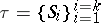is a decomposition ofinto parts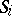that are images under the mapping (1) of sets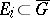forming a decompositionof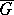(see Multiple integral) and if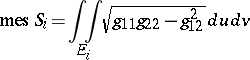is the area of, then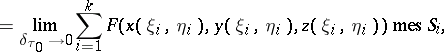where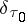is the fineness (mesh) ofand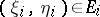. Ifis explicitly specified in the form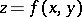,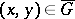, (2) becomesIf there are no singular points on a surfacewith vector representation (1), i.e. if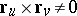, thencan be oriented by selecting a continuous unit normal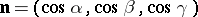on it, for exampleFor an oriented surfaceone defines the surface integrals of the second kind by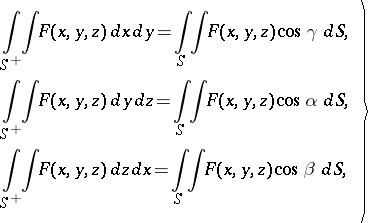(3)

where surface integrals of the first kind appear on the right-hand side. If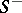is the surfacewith orientation opposite to that of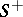, then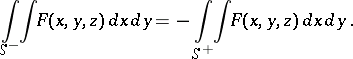Similar equations apply to the other surface integrals of the second kind in (3). Surface integrals of the second kind resemble those of the first kind in being the limits of Riemann sums, which can be described in terms of the surface.

If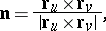then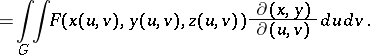Similar formulas apply to the other surface integrals of the second kind in (3).

In particular, for the case of a surface,,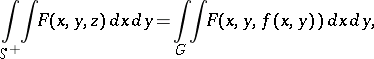The first of these integrals is called an integral over the "upper" side ofand the second over the "lower" side. This terminology is used because the vectorforms an acute angle with the-axis in the case of an explicit specification of, i.e. when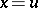,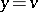and, i.e. it is directed "upwards" , while in the second case it forms an obtuse angle and is thus directed "downwards" .

If a smooth surfaceis the boundary of a bounded domain anddenotes its orientation by means of the outward normal, henceis determined by the inward normal, with respect to the domain, then the surface integrals of the second kind over the oriented surfaceare called surface integrals with respect to the outside of the surface, while those overare called surface integrals with respect to the inside.

The surface integrals for a piecewise-smooth surface that can be divided into a finite number of parts each of which has a vector representation (1) are defined as the sums of the surface integrals over the corresponding parts. Thus, surface integrals defined with respect to piecewise-smooth surfaces are not dependent on the method of dividing the surfaces into those parts.

The Ostrogradski formula establishes a relationship between a triple integral over a three-dimensional bounded domain and the surface integrals over its boundary, while the Stokes formula gives a relationship between a surface integral and the curvilinear integral over the contour representing the boundary.

The surface integral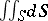is equal to the area of. If a mass with densityis distributed on, then the surface integral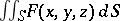is equal to the entire mass. If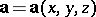is a vector function specified on a surfacethat is oriented by means of the unit normal, then the surface integral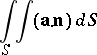is called the flux of the vector field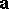through. Clearly, this is independent of the choice of a coordinate system in. One also uses surface integrals to describe a double-layer potential or a simple-layer potential.

If the surfaceis a differentiable-manifold, if the continuously-differentiable non-negative functions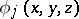,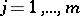, form a partition of unity on, i.e. the support of each function is contained in some chart ofand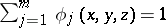for each point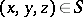, and ifis a function defined on, then by definition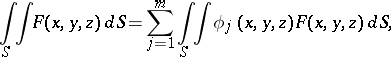(4)

where each integral on the right-hand side is understood in the sense of (2). Ifis an oriented two-dimensional manifold, then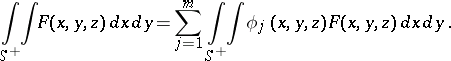(5)

The other types of surface integrals of the second kind in (3) are defined similarly. The definitions (4) and (5) are independent of the choice of a partition of unity in.

How to Cite This Entry:
Surface integral. Encyclopedia of Mathematics. URL: http://encyclopediaofmath.org/index.php?title=Surface_integral&oldid=18085
This article was adapted from an original article by L.D. Kudryavtsev (originator), which appeared in Encyclopedia of Mathematics - ISBN 1402006098. See original article